Ex 11.3

Chapter 11 Class 8 Mensuration
Serial order wise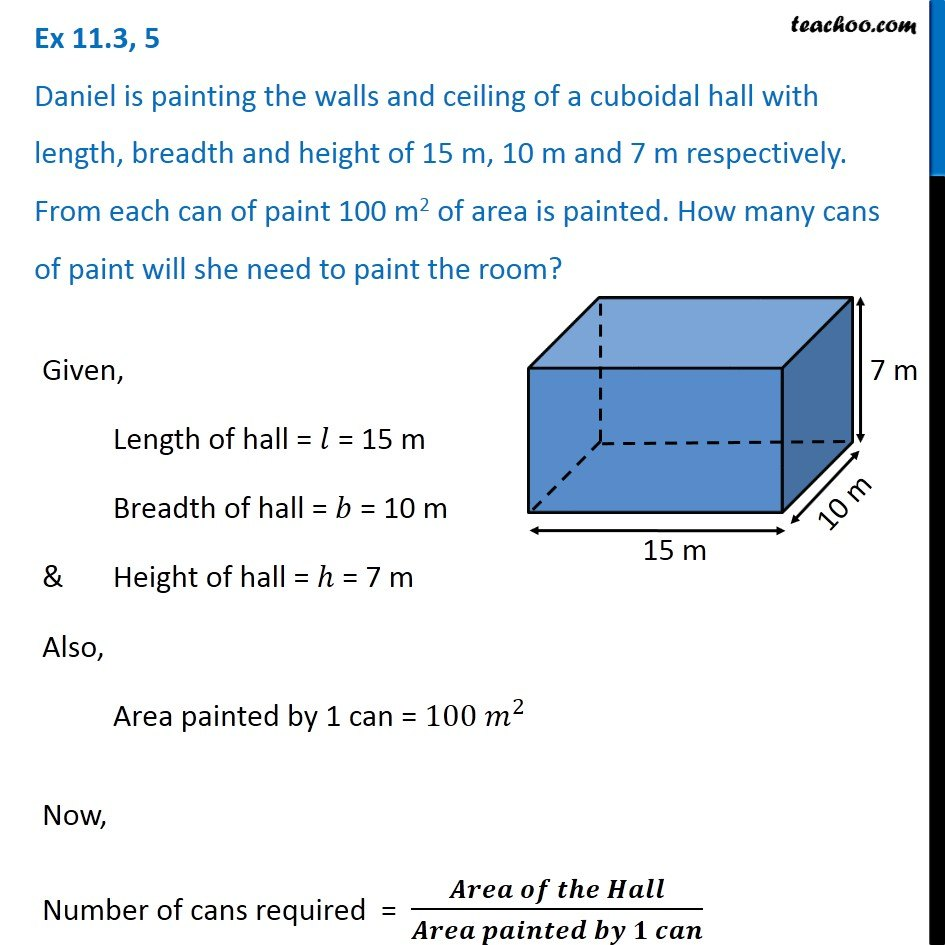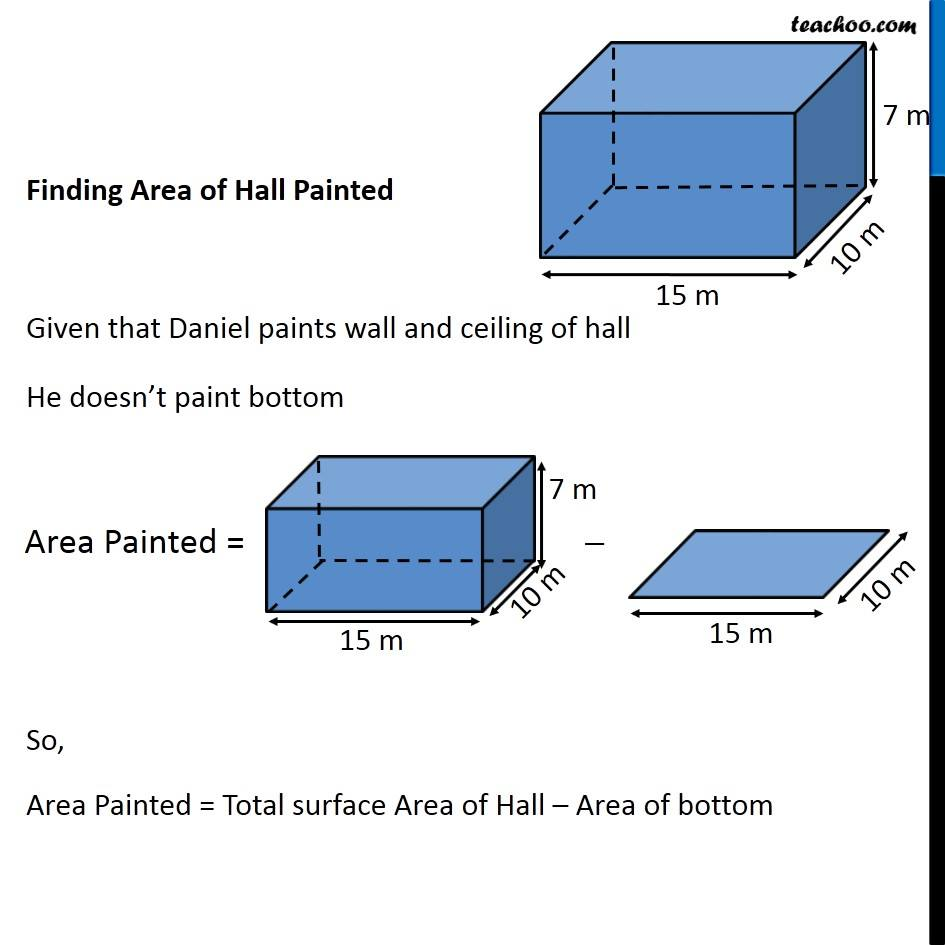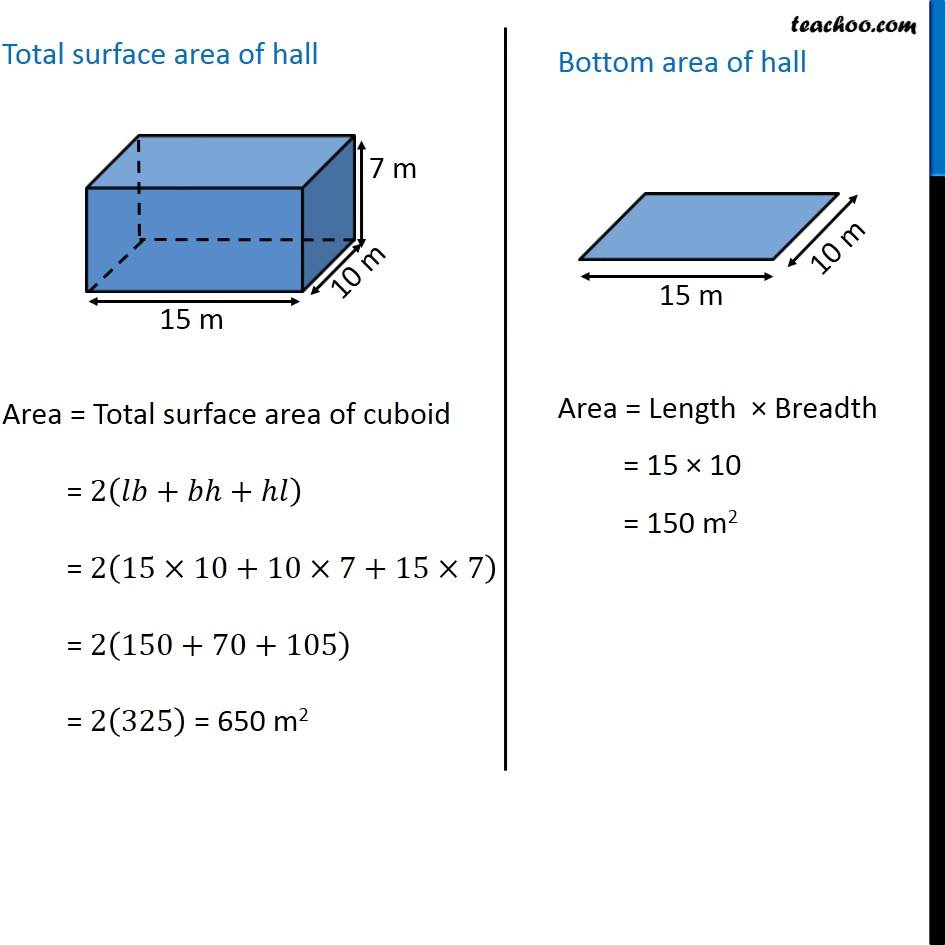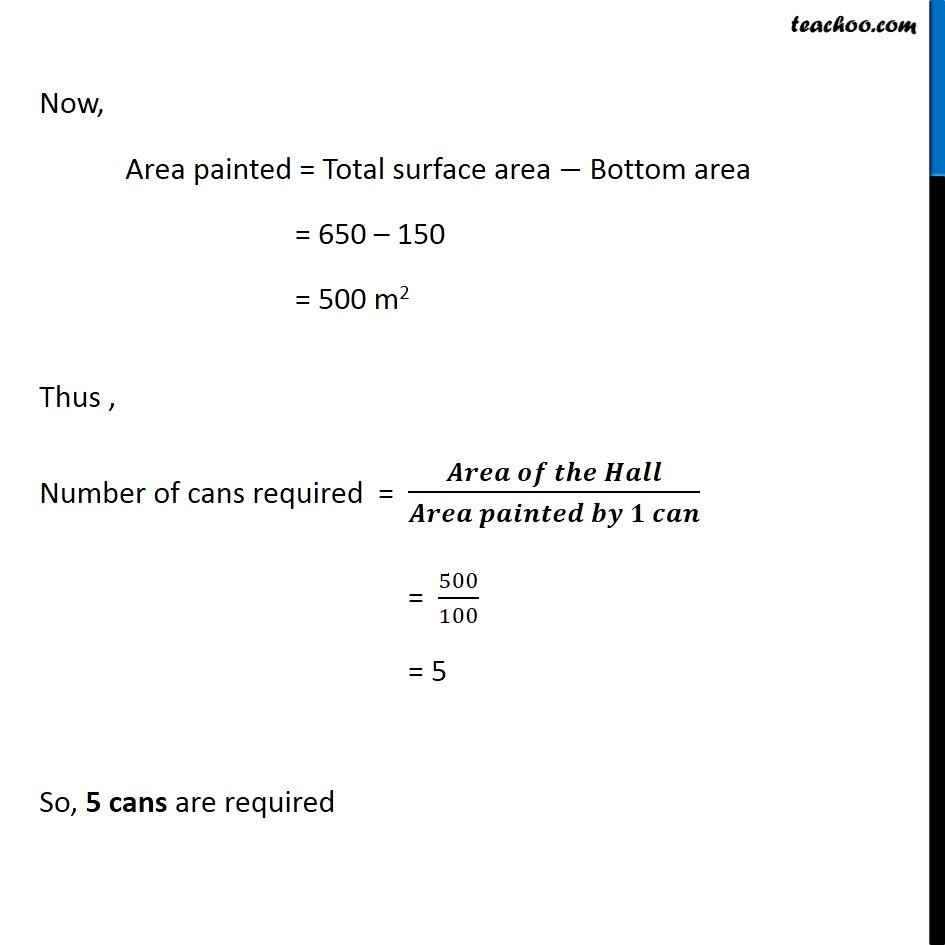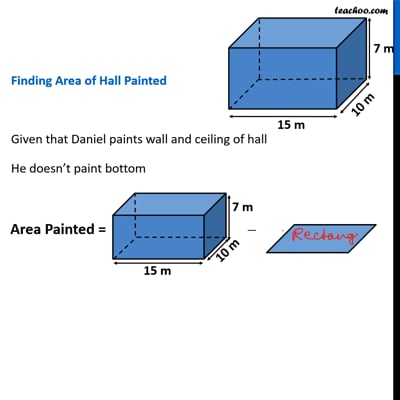This video is only available for Teachoo black users

Get live Maths 1-on-1 Classs - Class 6 to 12

### Transcript

Ex 11.3, 5 Daniel is painting the walls and ceiling of a cuboidal hall with length, breadth and height of 15 m, 10 m and 7 m respectively. From each can of paint 100 m2 of area is painted. How many cans of paint will she need to paint the room? Given, Length of hall = 𝑙 = 15 m Breadth of hall = 𝑏 = 10 m & Height of hall = ℎ = 7 m Also, Area painted by 1 can = 100 𝑚^2 Now, Number of cans required = (𝑨𝒓𝒆𝒂 𝒐𝒇 𝒕𝒉𝒆 𝑯𝒂𝒍𝒍)/(𝑨𝒓𝒆𝒂 𝒑𝒂𝒊𝒏𝒕𝒆𝒅 𝒃𝒚 𝟏 𝒄𝒂𝒏) Finding Area of Hall Painted Given that Daniel paints wall and ceiling of hall He doesn’t paint bottom So, Area Painted = Total surface Area of Hall – Area of bottom Total surface area of hall Area = Total surface area of cuboid = 2(𝑙𝑏+𝑏ℎ+ℎ𝑙) = 2(15×10+10×7+15×7) = 2(150+70+105) = 2(325) = 650 m2 Bottom area of hall Area = Length × Breadth = 15 × 10 = 150 m2 Now, Area painted = Total surface area − Bottom area = 650 – 150 = 500 m2 Thus , Number of cans required = (𝑨𝒓𝒆𝒂 𝒐𝒇 𝒕𝒉𝒆 𝑯𝒂𝒍𝒍)/(𝑨𝒓𝒆𝒂 𝒑𝒂𝒊𝒏𝒕𝒆𝒅 𝒃𝒚 𝟏 𝒄𝒂𝒏) = 500/100 = 5 So, 5 cans are required